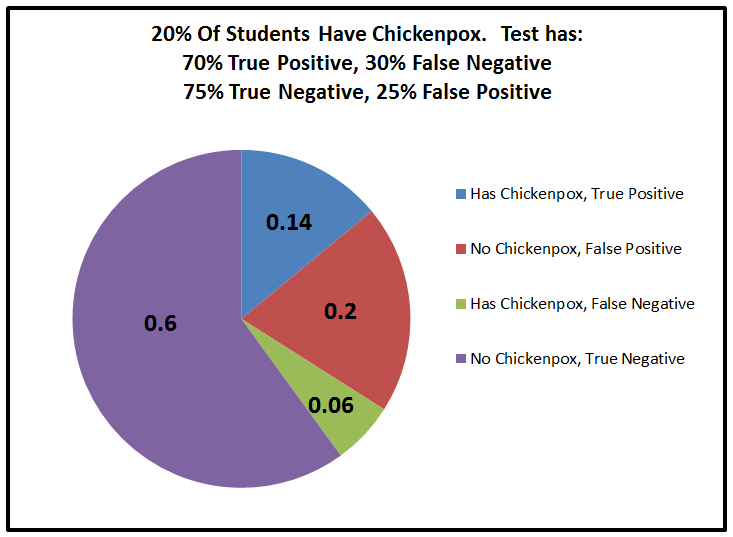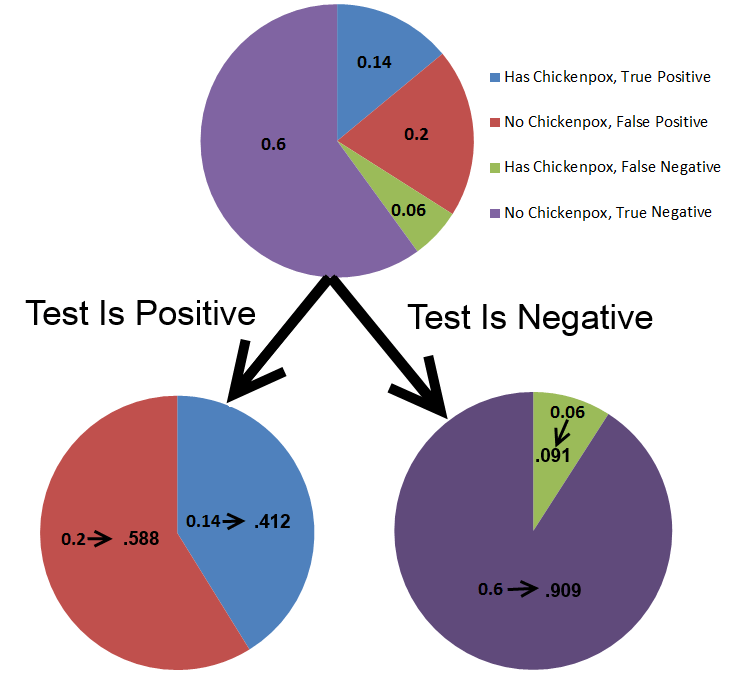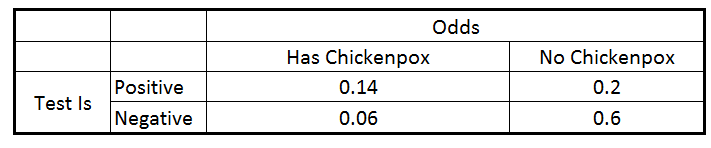# Bayes Theorem Summary

For the basics of Bayes Theorem, I recommend reading my short introductory book “Tell Me The Odds” It is available as a free PDF or as a Free Kindle Download, and only about 20 pages long, including a bunch of pictures.  It will give you a great understanding of how to use Bayes Theorem.

What Is Bayes Theorem – In 3 Sentences

Bayes Theorem is a way of updating probability estimates as you get new data.  You see which outcomes match your new data, discard all the other outcomes, and then scale the remaining outcomes until they are a full 100% probability.

Bayes Theorem As An Image

Medical Testing is a classic Bayes Theorem Problem.   If you know 20% of students have chickenpox, and you test every student with a test that gives 70% true positive, 30% false negative when they have chickenpox and 75% true negative, 25% false positive when they don’t.   Then before doing the test, you can construct a probability table of the 4 possible outcomes.This is not Bayes Theorem.  This is just a probability table.  Bayes Theorem is used when you get new data, eliminate some of the possible outcomes, and scale the other ones back up to 100% probability.  Shown belowWhen Is Bayes Theorem Used?

• The applications of Bayes Theorem are far ranging.
• It is commonly used in medical testing. For instance, you might make an initial estimate of your risk of heart disease based on the average rate of the disease in people your age, but then revise that risk once you receive new relevant information, such as your blood pressure or cholesterol results.
• Bayes Theorem was one of the first successful spam filters. You can estimate the odds that an email is spam, and then revise that estimate based on how spammy each word in the email is
• Bayes Theorem has been used to locate lost airplanes, based on what search results have turned up.

Key Points

• Bayes Theorem is just multiplication and division, with a choice of which probabilities to use
• The easiest way to think of Bayes theorem is that it is two probabilities in sequence. So list out all possible pairings of probabilities, such as in a table• And then discard any that don’t match the observed new data• And then scale the remaining probabilities until they sum to 1.0• Here is a blog post that goes into deeper detail for a medical testing problem
• Probabilities can be updated multiple times based on multiple new observations. This can be done  by doing this process multiple times in series
• One thing to be aware of is to never set any probability to zero unless you are absolutely certain it cannot occur
• This SMBC comic gives an example: Bayesian Vampire
• This XKCD comic gives an example of not taking into account the very low value of the prior, i.e. just focusing on the likelihood. Sun Exploding
• If you set a probability to zero, it will be zero forever. If you set it to a really low number, it can come back if a bunch of later observations indicate that you were in error to set it so low.
• I have a Kindle book with a number of example problems for Bayes Theorem
• A more detailed and math heavy, but very good, book is “Think Bayes”.  This is a good choice if you are familiar with Python programming

2nd Level

• Many more complicated problems start to combine Bayes Theorem with different probability distributions
• For instance, instead of assuming a uniform probability distribution for the prior, a normal distribution might be a better approximation. These refinements are problem specific depending on what probability distribution best represents the problem at hand.
• To figure out which possibilities are getting more or less probable, you can take the ratio of their likelihoods based on any given observation. This is the called “Bayes Ratio” or the “Likelihood Ratio”
• More data is a great thing. Enough data can overwhelm any approximation you made in your initial estimation of probabilities (i.e. the prior) or in your likelihood calculation.
• The easiest way to think about this is that a final probability is the product of the initial probability with all of the Bayes Adjustments from each new observation
• Prior * #1 * #2 * #3
• Eventually, the final probability is going to be dominated by all of the new observations rather than the prior